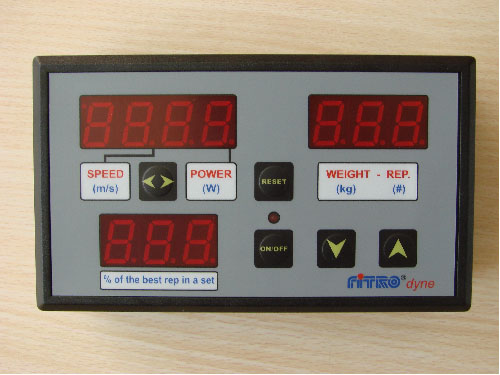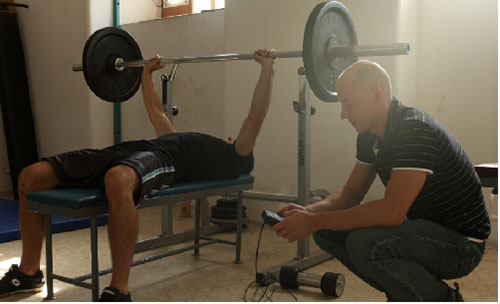A portable system for the assessment of strength capabilities and feed back control of the weight training.

## Construction and principle of operation

The system consists of sensor unit and small microcomputer. The encoder based sensor unit mechanically coupled with the barbel by means of kevlar tether monitors the velocity of the weight lifted and microcomputer calculates mean and peak power in concentric phase of exercise.

Pulling the tether (connected by means of small hook either to barbell axis or to the weight lifted) rotates the reel with connected velocity sesor (encoder). Reel is rewinded by a string producing force of about 2 N. The tether can be pulled out up to 2.5 meter. Signal from sensor unit is conveyed to the microcomputer.

The calculations are based on the Newton´s law of universal gravitation (force equals mass multiplied by gravitational constant) and the Newton´s law of motion (force equals mass multiplied by acceleration).

Instant force while moving barbell of the mass m in vertical direction is calculated as a sum of gravitational force (mass multiplied by gravitational constant) and acceleration force (mass multiplied by acceleration).

Acceleration of upward vertical movements is obtained by derivation of velocity. Instant power is calculated as a product of force and velocity.

FiTROdyne Basic comes in 3 models with different display units.

## FiTROdyne Basic Peak Power LCD

Control unit features LCD display. The unit can store the data from up to 2000 repetitions, which can be transferred to the PC for further storage and processing using special software.
Alternatively, data can be collected directly by simultanous PC connection.

## FiTROdyne Basic Peak Power LED

Control unit based on larger LED display providing better feedback information to exercising subject. The unit can store the data from up to 2000 repetitions, which can be transferred to the PC for further storage and processing using special software.
Alternatively, data can be collected directly by simultanous PC connection.## FiTROdyne Basic PC

System consists of sensor unit and user´s own PC providing mean values of velocity and force from concentric phase. In addition to convenient storage of the data, the system also allows extensive reporting in digital as well as in graphical form including force/velocity and power/velocity graphs based on the data collected in diagnostic series.